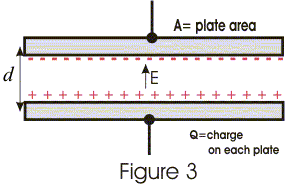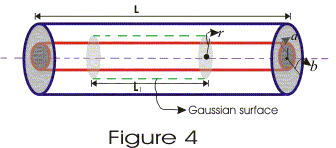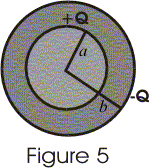# Capacitance

## 3. Parallel plate capacitor

• A parallel plate capacitor consists of two large plane parallel conducting plates separated by a small distance shown below in the figure 3.• Suppose two plates of the capacitor has equal and opposite charge Q on them. If A is the area of each plate then surface charge density on each plate is
σ=Q/A
• We have already calculated field between two oppositely charged plates using gauss's law which is
E=σ/ε0=Q/ε0A
and in this result effects near the edges of the plates have been neglected.
• Since electric field between the plates is uniform the potential difference between the plates is
V=Ed=Qd/ε0A
where , d is the separation between the plates.
• Thus, capacitance of parallel plate capacitor in vacuum is
C=Q/V=ε0A/d               (3)
• From equation 3 we see that quantities on which capacitance of parallel plate capacitor depends i.e.,ε0 , A and d are all constants for a capacitor.
• Thus we see that in this case capacitance is independent of charge on the capacitor but depends on area of it's plates and separation distance between the plates.

## 4.Cylinderical capacitor

• A cylinderical capacitor is made up of a conducting cylinder or wire of radius a surrounded by another concentric cylinderical shel of radius b (b>a).

• Let L be the length of both the cylinders and charge on inner cylender is +Q and charge on outer cylinder is -Q.
• For calculate electric field between the conductors using Gauss's law consider a gaussian consider a gaussian surface of radius r and length L1 as shown in figure 4.• According to Gauss's law flux through this surface is q/ε0 where q is net charge inside this surface.
• We know that electric flux is given by
φ=E.A
=EAcosθ
=EA

since electric field is constant in magnitude on the gaussian surface and is perpandicular to this surface. Thus,
φ=E(2πrL)
since            φ=q/ε0
=>           E(2πrL)=(λL)/ε0

where λ = Q/L = charge per unit length
=>           $$E=\frac{\lambda }{2\pi \epsilon _{0}r}$$
(4)
• If potential at inner cylinder is Va and Vb is potential of outer cylinder then potential difference between both the cylinders is
V=Va and           Vb=∫Edr
where limits of integration goes from a to b.

• Potential of inner conductor is greater then that of outer conductor because inner cylinder carries positive charge. Thus potential difference is $$V=\frac{Qln(b/a)}{2\pi \epsilon _{0}L}$$
• Thus capacitance of cylinderical capacitor is
C=Q/V
or, $$C=\frac{2\pi \epsilon _{0}L{(b/a))}$$
(5)
• From equation 5 it can easily be concluded that capacitance of a cylinderical capacitor depends on length of cylinders.
• More is the length of cylinders , more charge could be stored on the capacitor for a given potential difference.

## 5. Spherical capacitor

• A spherical capacitor consists of a solid or hollow spherical conductor of radius a , surrounded by another hollow concentric spherical of radius b shown below in figure 5• Let +Q be the charge given to the inner sphere and -Q be the charge given to the outer sphere.
• The field at any point between conductors is same as that of point charge Q at the origin and charge on outer shell does not contribute to the field inside it.
• Thus electric field between conductors is $$E=\frac{Q}{2\pi \epsilon _{0}r^{2}}$$

• Potential difference between two conductors is
V=Va-Vb
=-∫E.dr

where limits of integration goes from a to b.
On integrating we get potential difference between to conductors as
$$V=\frac{Q(b-a)}{4\pi \epsilon _{0}ba}$$
• Now , capacitance of spherical conductor is
C=Q/V
or, $$C=\frac{4\pi \epsilon _{0}ba}{(b-a)}$$
(6)
• again if radius of outer conductor aproaches to infinity then from equation 6 we have
C=4πε0a          (7)
• Equation 7 gives the capacitance of single isolated sphere of radius a.
• Thus capacitance of isolated spherical conductor is proportional to its radius.